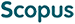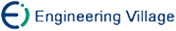卢友军, 许道云. 随机图中k-独立集的相变性质[J]. 计算机研究与发展, 2017, 54(12): 2843-2850.
 引用本文: 卢友军, 许道云. 随机图中k-独立集的相变性质[J]. 计算机研究与发展, 2017, 54(12): 2843-2850.Lu Youjun, Xu Daoyun. Phase Transition Properties of k-Independent Sets in Random Graphs[J]. Journal of Computer Research and Development, 2017, 54(12): 2843-2850.
 Citation: Lu Youjun, Xu Daoyun. Phase Transition Properties of k-Independent Sets in Random Graphs[J]. Journal of Computer Research and Development, 2017, 54(12): 2843-2850.## Phase Transition Properties of k-Independent Sets in Random Graphs

• 摘要: 相变性质是ER(Erds-Rényi)随机图理论具有的重要性质，一个简单无向图G=(V,E)中的k-独立集是一个具有k个顶点的独立集.为更好地理解ER随机图中k-独立集的结构特性，提出并利用一阶矩和二阶矩方法严格证明了当2≤k=ο(n)时随机图G(n,p)中k-独立集出现相变的临界概率p\-c=1－n\+－2/k－1.利用m≈pC\+2\-n时随机图G(n,p)和G(n,m)等价的性质给出了随机图G(n,m)中k-独立集出现相变的临界边数m\-c=［n(n－1)/2(1－n\+－2/k－1)］.实验结果表明:当2≤k=ο(n)时，随机图G(n,p)和G(n,m)中存在k-独立集的理论临界值和仿真得到的临界值一致且临界值与图节点总数n和独立集节点数k有关，而当k=ω(n)时，随机图G(n,p)和G(n,m)中存在k-独立集的理论临界值和仿真临界值不一致.

Abstract: Phase transition property is one of the most important properties of the theory of Erds-Rényi random graphs. A subset of vertices is a k-independent set in a simple undirected graph G=(V,E) if the subset is an independent set containing k vertex. In order to understand the structural property of k-independent sets in Erds-Rényi random graphs, the phase transition properties of k-independent sets in Erds-Rényi random graphs are investigated in this paper. It is shown that the threshold probability is p\-c=1－n\+－2/k－1 for the existence of k-independent sets in random graph G(n,p) via the first moment method and the second moment method when 2≤k=ο(n). According to this fact that random graph G(n,p) is equivalent to random graph G(n,m) when m is close to pC\+2\-n, the threshold edge number is given by m\-c=［n(n－1)/2(1－n\+－2/k－1)］ for the existence of k-independent sets in random graph G(n,m). The simulation results show that the consistence between simulation and theoretical threshold value for the existence of k-independent sets in random graph G(n,p) and G(n,m) when 2≤k=ο(n), and the threshold value is related to the total number n of vertices and the number k of vertices of independent set. However, when k=ω(n), the theoretical threshold value is not consistent with the simulation threshold value for the existence of k-independent sets in random graph G(n,p) and G(n,m)./下载:  全尺寸图片 幻灯片
• 分享
• 用微信扫码二维码

分享至好友和朋友圈